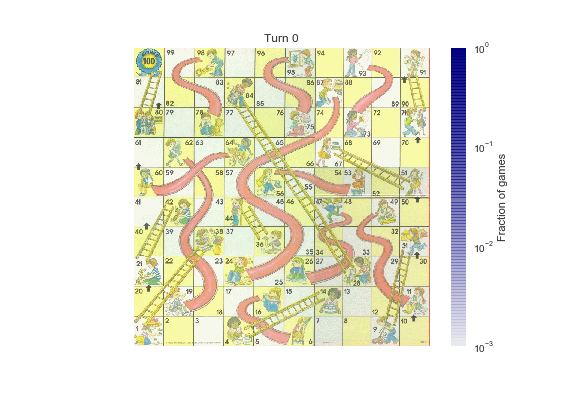# Snakes and Ladders can be analyzed by converting it to a Markov ChainUniversity of Washington data scientist Jake Vanderplas found himself trapped in an interminable series of Snakes and Ladders (AKA Chutes and Ladders) with his four-year-old and found himself thinking of how he could write a Python program to simulate and solve the game.

After some keyboard tinkering, he simulated the whole game as an Absorbing Markov Chain, which turned out to be surprisingly elegant, and yielded some interesting insights into the game's underlying logic: it can be finished in as few as seven moves, while the median game requires 32 moves per player (the mode is a complete game in 22 moves); the least-likely square is 67.

From the first square, the expected time to completion is 39.7 moves: this is half a move than the 39.2 moves we computed previously, because we're using a different form of the matrix (here we count climbing the ladder from square 80 to square 100 as one extra move)

This vector can tell us what the best first roll is: as my daughter has intuited, starting out by rolling a 1 and climbing the first ladder is best: it decreases your expected time to completion by five moves. The worst first roll is a 2: this actually increases your expected time to completion by 0.5 turns, on average: rolling a 2 to start is akin to moving away from the goal.

By the time you get to the 99th square, your espected time to completion is 6 moves, because you have a 1/6 chance of rolling the 1 required to win. Two squares back, on the 97th square, your expected time to completion goes up to 11 moves, because of the chute present on the 98th square.

The fundamental matrix lets you quantititatively explore a number of other properties of the game; for example, we could adjust the matrix to make square 80 an absorber as well, and ask how probable we are to complete the game there versus landing on square 100 directly. Or we could compute quantities such as the variance of the number of visits and number of steps above. For more discussion of applications of the fundamental matrix of an absorbing Markov chain, see the wikipedia article. A nice undergraduate-level introduction to these concepts can be found in Chapter 11 of Grinstead and Snell's “Introduction to Probability” (pdf available here).

Simulating Chutes & Ladders in Python [Jake Vanderplas/Github]